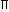As an example of this memory technique for memorising two equations like these =

1. Curved surface area of a cylinder = (2rh ) sq. units.
2. Total surface area of a cylinder = 2r ( h + r ) sq. units.
And here it can be seen that the length of the equation of the CSA of a cylinder is smaller than that the length of the equation of the TSA of a cylinder and the length of the equation of the TSA of the cylinder is greater than the CSA of the cylinder and for this.It can be imagined that there is a family of two people of a father and his son and the father as an adult,whose height is bigger or greater than his child gets a rotated 2 put on his head so that it represents an alphabet(which in this case is “n”).And then he says “piraah”(pi=,raah=memonic of rh) while his son being smaller than his father gets an gets a rotated 2 put on his head so that it represents an alphabet(which in this case is “n”) again.and then says “pihaar”( pi=,haar(A hindi word)=memonic of hr) and both of them can put a different resembling object in an orederd labled box just like the in the shaper system-https://forum.artofmemory.com/t/the-shaper-system-number-shapes-00-99-anyone-using-it/33221 and optionally each sub-box can also be locked and can made to open by making the people in this system hold objects as keys respectively to open the box(In this case a very high tech box with a camera) and then retrive a single object or even multiple objects after opening the box and by using the journey method along with the different prespectives of each character this method can be made even more powerful although very tiring,and credits to all the members of this form and the people whose ideas I have used to make this system(Note: To be fair to other people whose names I have not mentioned here I will also not disclose my real name in this form and I will also not take most of the credit for this system.),What are your thoughts about this system,Any comments will be appreciated,Cheers.
4 Likes

You can remember this type of formula easily with the help of concepts.

I can show you this type of formula with the help of daily life objects .
First we imagine we put an bangle on the table (Area of circle) and we put more bangles on previous bangles.
So it becomes high (means h )
And it becomes cylinder like structure.

So, Volume of cylinder = bangles * it’s height
= Area of circle * h
= πr^2h

Note = if you don’t know the formula of area of circle then you imagine an Pizza
Pi = π
zz = rr = r^2 ( imagine z is radius)
A = it gives you hint that this is the formula of
area of circle.

And when you understood this than you can easily remember the formula of volume of come.

Volume of Cone = 1/3 πr^2h

Imagine you cut cylinder in three equal pieces so it can easily remember 1/3 . And rest formula is same.

CSA of cylinder = perimeter of circle * h

And if you want only mnemonic to Learn.
I give you one example of CSA of Cone

CSA of Cone = πrl ( imagine we put PARLE G in cone)

Parle g biscuits = πrl

2 Likes

Well,I had tried this method before but this could not work for me.

Its nice.
Can anyone help in this.

Price elasticity is equal to percentage change in quantity demanded upon percentage change in price.
Price Elasticity(Ed) = (Percentage change in quantity demanded)/(Percentage change in Price), Ed = (%ΔQ)/(%ΔP) or Ed = (ΔQ/Q)/(ΔP/P)
Ed= Price Elasticity
Q=Original Quantity demanded
P= Original Price of the Commodity
ΔQ= Change in quantity demanded (New demand - Original demand)
ΔP=Change in Price(New Price-Original Price)

I think it is the easiest formula.
You can easily remember this with my way.

Price elasticity = means People are fighting for money.

Whenever people fighting for money then it is the beginning of war.

In war people started to fight with Cannon (%) and throw in triangular shape above the sky a Bomb (Q).
And in below By Cannon Person ( P ) are dieing.

Price elasticity = %∆Q (Cannon triangular shape bomb) / %∆P (Cannon triangular shape person)

Note : ( / ) it means The surface of road.

This is only the way to memorize easily but I also suggest you that Understand the whole formula with their meaning and try to apply in real life. It is the perfect way to remember formula.

1 Like